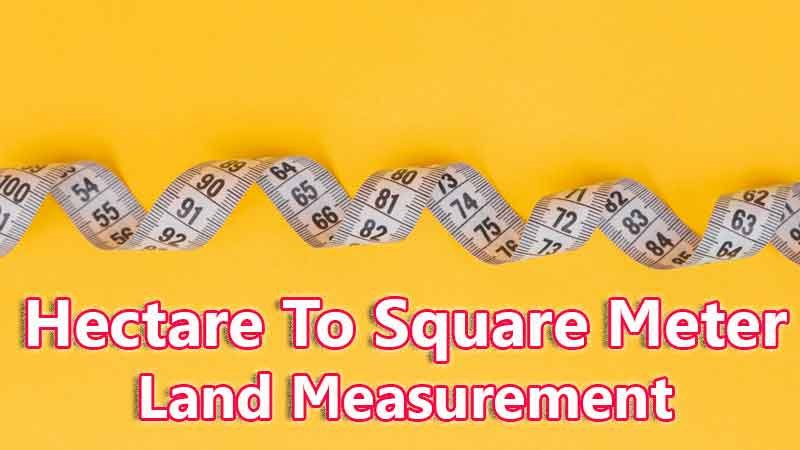# Hectare To Square Meter Converter

Hectare is a traditional land unit used to measure the land Area standardly. Here we are using the hectare to square meter unit value conversion. And hectare also comes under these land area units like Gonda, Kani, and square meter. In West Bengal, One hectare is directly equivalent to 10000 square meter.

Input Here

hectare

Output

square meter

1 hectare = 10000 square meter

## Measurement

Hectare means - a unit of land measurement. This tool provides great calculation over hectare to square meter, 1 hectare to square meter, 1 hectare in square meter, 1 hectare equal to and etc. To convert hectare to square meter, then multiply the unit value by 10000. Then, 1 hectare * 10000 = 10000 square meter. Hence, 1 hectare is equal to 10000 square meter.

Square Meter also comes under this land measurement unit and we are comparing values between hectare traditional unit with other side land measurement unit.

## Formula For hectare to square meter Conversion(hectare to square meter formula)

The base formula for this hectare to square meter converter is

square meter = hectare * 10000

## How many square meter in a hectare?

Step 1: To Convert 1 hectare to square meter

Step 2: Applying formula square meter = hectare * 10000, (i.e) multiply the unit value by 10000.

Step 3: Then, square meter = 1 * 10000 = 10000.

Step 4: Hence, 1 hectare is equal to 10000 square meter.Some hectare to square meter Conversion Chart for your reference:

 1 hectare = 10000 square meter 2 hectare = 20000 square meter 3 hectare = 30000 square meter 4 hectare = 40000 square meter 5 hectare = 50000 square meter 6 hectare = 60000 square meter 7 hectare = 70000 square meter 8 hectare = 80000 square meter 9 hectare = 90000 square meter 10 hectare = 100000 square meter

The hectare to square meter conversion chart is above listed for your reference. This chart, however, represents the simple math calculation involved in the hectare to square meter convertion online.

For Example: How much is 1 hectare to square meter

Solution:

= (hectare * 10000)

= (1 x 10000)

= 10000 square meter

For Example: How much is 120 hectare in square meter

Solution:

= (hectare * 10000)

= (120 x 10000)

= 1200000 square meter

This formula provides an instant answer for all your questions / People also search:

• 1 hectare to square meter
• 1 hectare is equal to how many square meter
• Formula to convert hectare to square meter online
• How to convert hectare to square meter?
• How much square meter is equal to a one hectare?
• How many square meter in hectare?
• 1 hectare equal to square meter